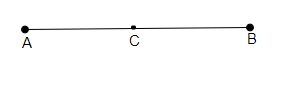# If a point $C$ lies between two points $A$ and $B$ such that $A C=B C$, then prove that $\mathrm{AC}=\frac{1}{2} \mathrm{AB}$. Explain by drawing the figure.

Given:

A point $C$ lies between two points $A$ and $B$ such that $AC=BC$.

To do:

We have to prove that $AC=\frac{1}{2}AB$.

Solution:Given,

$AC=BC$

By adding $AC$ on both sides we get,

$AC+AC=BC+AC$

This implies,

$2AC=BC+AC$    ($BC+AC$ coincides with $AB$)

According to Euclid's Axiom $4$

$BC+AC=AB$.

Therefore,

$2AC=AB$

This implies,

$AC=\frac{1}{2}AB$

Updated on: 10-Oct-2022

15 Views